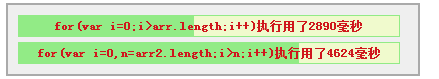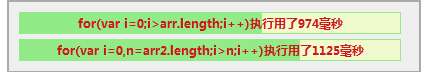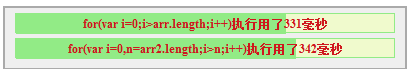javascript技巧# js 数组的for循环到底应该怎么写？

var arr=[],arr2=[],i=0
while(i<100000){
arr.push(i)
arr2.push(i)
i++
}
M.TA.begin("0000");
for(var i=0;i<arr.length;i++){
arr[i]=arr[i]*arr[i]*arr[i]
}
M.TA.end("0000","for(var i=0;i<arr.length;i++)")
M.TA.begin("0001");
for(var i=0,n=arr2.length;i<n;i++){
arr2[i]=arr2[i]*arr2[i]*arr2[i]
}
M.TA.end("0001"," for(var i=0,n=arr2.length;i<n;i++)")
M.TA.showResult()

chromefirefox（在做过N种性能测试后发现firefox在基础运算上的效率比chrome高很多，只是涉及到渲染的就慢很多了）
ie8（减少了N个数量级之后的记过，上述脚本在ie里无法运行）

http://www.html-js.com